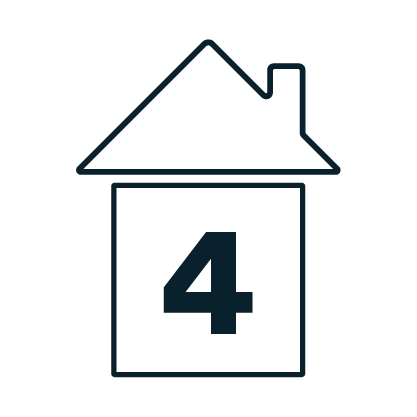##Grade 4 Module 5 Lesson 21

How can we use tape diagrams, number lines, and number bonds to add fractions? Join Ms. DelFavero for this lesson where weâll use mathematical models to add fractions with related units. For this lesson, youâll need paper and pencil. If you have access to a workbook, or a printer, we also suggest using the Problem Set available using the link below the video